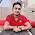Program:
class Area
{
int a,b;
void area(int a)
{
double A=3.14*a*a;
System.out.print("\n\tArea of circle="+A);
}
void area(int a,int b)
{
double B=a*b;
System.out.print("\n\tArea of Rectangle="+B);
}
}
{
public static void main(String args[])
{
Area A1=new Area();
A1.area(10);
A1.area(5,10);
}
}

Output:
nn@linuxmint ~ \$ javac methodoverload.java
nn@linuxmint ~ \$ java methodoverload

Area of circle=314.0
Area of Rectangle=50.0
nn@linuxmint ~ \$

1.2.3.